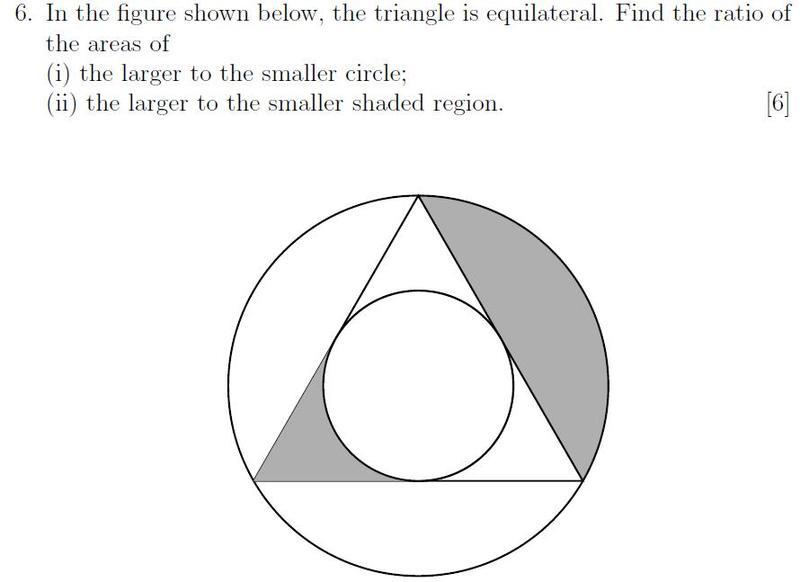# Circle problem

david18
I'm having trouble with this question. For the first part Im guessing that the radius is 2 times larger for the bigger circle and therefore its area is four times as large, but I can't seem to prove that the radius is twice as large.

I'd appreciate it if anyone could help me out on this question## Answers and Replies

dirk_mec1
If you want a quick answer upload your image on imageshack...

Homework Helper
No. The radius of the large circle is NOT twice the radius of the small circle. To find the ratio, Assume the length of each side of the equilateral triangle is L. If you drop a perpendicular from one vertex to the opposite side, it bisects that side. You now have a right triangle with hypotenuse of length L and one leg of length L/2. Letting "x" be the length of the other leg, by the Pythagorean theorem, you have L2= x2+ L2/4 or x2= 3L2/4. x= $\sqrt{3}/2$. Now draw another line from another vertex to the center of the circle. Then you have another right triangle with hypotenuse of length R, the circumference of the circle, one leg of length L/2 and the other of length $\sqrt{3}/2- R$. Now the Pythagorean theorem gives $R^2= L^2/4+ ((\sqrt{3}/2)L- R)^2$ which reduces to $L^2/4+ (3/4)L^2- \sqrt{3}Lh= 0$ (the "R^2" terms cancel) so $R= L/\sqrt{3}= \sqrt{3}L/3$.

Remember that the first vertical line has length $\sqrt{3}L/2$. We now see that can be divided into a short distance of $\sqrt{3}L/3$ and a longer distance of $\sqrt{3}L/2- \sqrt{3}L/2= \sqrt{3}L/6$. The length of the longer part is the radius of the larger circle, and the length of the shorter part is the radius of the smaller circle.
$$\frac{\frac{\sqrt{3}}{2}L}{\frac{\sqrt{3}}{6}L}= 3$$
not 2.

david18
thanks for the reply.

I was working through your solution and knowing the context of the question, thought it seemed a little too complicated.

What I eventually found is that if you draw a line from the centre to a corner of the triangle, and another line from the centre to the tangent, you form a right angled triangle with angles of 30 and 60 degrees. The line from the centre to the tangent is r, so knowing that sin 30 = 1/2, you say that the hypotenuse must be 2r.

Sorry for the poor explanation, I can draw up a solution if you have any trouble going through the lengthy explanation.